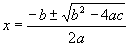The most useful (but not always the easiest to use) formula for solving quadratics is the quadratic formula. In order to use it, the equation must be in the standard formNote that this standard form differs from that used with completing the square (CTS from now on), so it is important to work with the equations and their respective standard forms so much that confusion is unlikely. We will use completion of the square and a little substitution to derive the quadratic formula. First, divide the equation by a:Next, substituteAnd the equation becomesThis second version of the equation is in standard form for CTS (compare them and see how different they are). Plugging the appropriate symbols into the CTS formula givessolving for x givesUnsubstituting b and g givesNote that we multiplied everything under the radical by a2 and divided outside the radical by a. Rearranging this just a little gives us